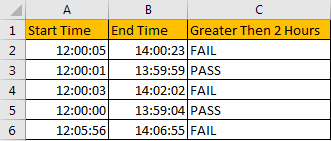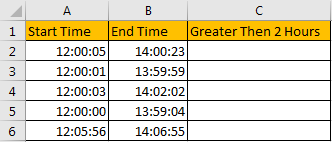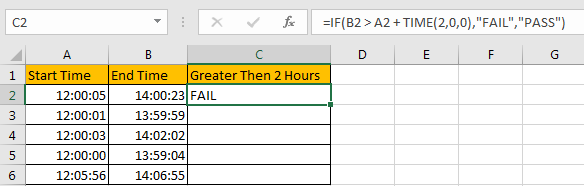# How to Compare Time Difference with Certain Time in Excel

Sometimes we need to count time difference when participating a contest or an exam, and if the time difference (between start time and end time) is greater than certain time, participants are failed in the contest. So, in this situation we need to know the method to compare time difference with certain time. This article will introduce you a quick method to fix this issue.

Precondition:

Prepare a table record the start time and end time of a contest. And if time difference is greater than 2 hours, participators fail the contest, on the other side, if time difference is less than 2 hours, they pass the contest. We need to enter PASS or FAIL in column C.## Method: Compare Time Difference with Certain Time in Excel

Step 1: In C2 enter the formula =IF(B2 > A2 + TIME(2,0,0),”FAIL”,”PASS”). In this formula, if B2 is greater than A2+2 hours, then formula returns FAIL, otherwise it returns PASS.Step 2: Drag the fill handle down to fill B3:B6. Verify that B3:B6 are filled with FAIL or PASS.We can see that in this example, only two people pass the contest.

Comment:

1. As TIME(HOUR,MINUTE,SECOND), so we can change hour/minute/second values per our real demands. If we want compare time difference with 2 hour 5 minutes 10 seconds, we can update the formula as =IF(B2 > A2 + TIME(2,5,10),”FAIL”,”PASS”).

### Related Functions

• Excel TIME function
The Excel TIME function returns a date in serial number format for a particular time (the hour, minute and second).The syntax of the TIME function is as below:= TIME(hour, minute, second)…
• Excel IF function
The Excel IF function perform a logical test to return one value if the condition is TRUE and return another value if the condition is FALSE. The IF function is a build-in function in Microsoft Excel and it is categorized as a Logical Function.The syntax of the IF function is as below:= IF (condition, [true_value], [false_value])….